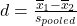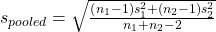Hypothesis Testing with Two Samples

# 50 Cohen’s Standards for Small, Medium, and Large Effect Sizes

<!– Insert module text here –>Cohen’s d is a measure of “effect size” based on the differences between two means. Cohen’s d, named for United States statistician Jacob Cohen, measures the relative strength of the differences between the means of two populations based on sample data. The calculated value of effect size is then compared to Cohen’s standards of small, medium, and large effect sizes.

Cohen’s Standard Effect Sizes
Size of effect d
Small 0.2
Medium 0.5
Large 0.8

Cohen’s d is the measure of the difference between two means divided by the pooled standard deviation:whereIt is important to note that Cohen’s d does not provide a level of confidence as to the magnitude of the size of the effect comparable to the other tests of hypothesis we have studied. The sizes of the effects are simply indicative.

Calculate Cohen’s d for (Figure). Is the size of the effect small, medium, or large? Explain what the size of the effect means for this problem.

1 = 4 s1 = 1.5 n1 = 11

2 = 3.5 s2 = 1 n2 = 9

d = 0.384

The effect is small because 0.384 is between Cohen’s value of 0.2 for small effect size and 0.5 for medium effect size. The size of the differences of the means for the two companies is small indicating that there is not a significant difference between them.

### Chapter Review

Cohen’s d is a measure of “effect size” based on the differences between two means.

It is important to note that Cohen’s d does not provide a level of confidence as to the magnitude of the size of the effect comparable to the other tests of hypothesis we have studied. The sizes of the effects are simply indicative.

### Formula Review

Cohen’s d is the measure of effect size:where